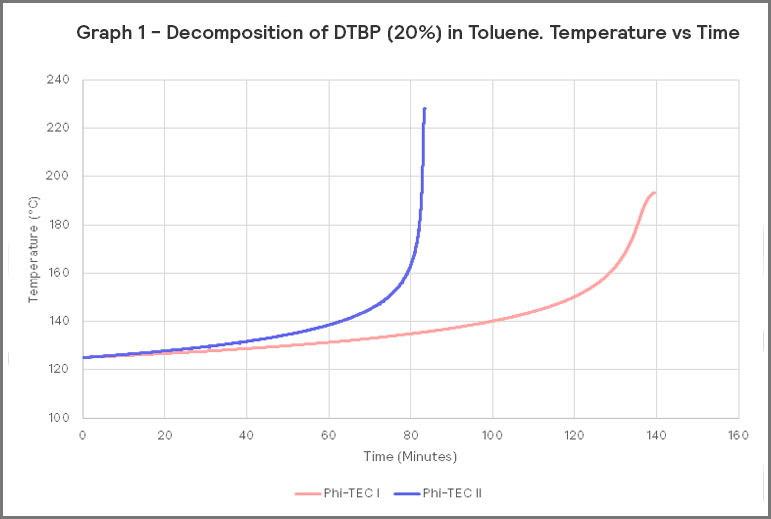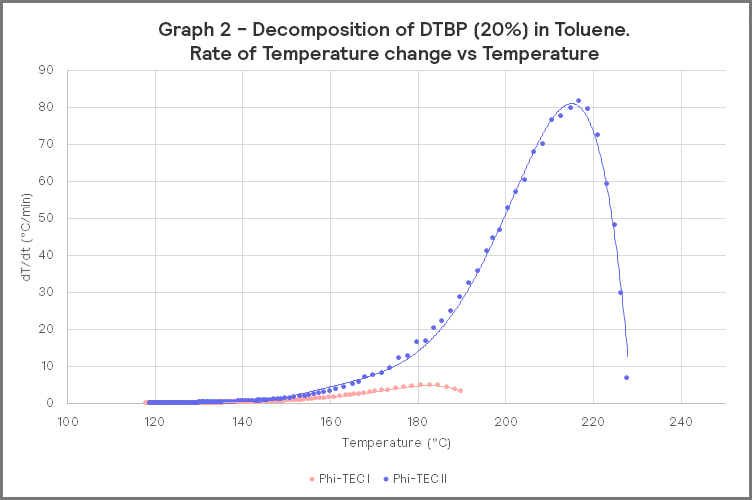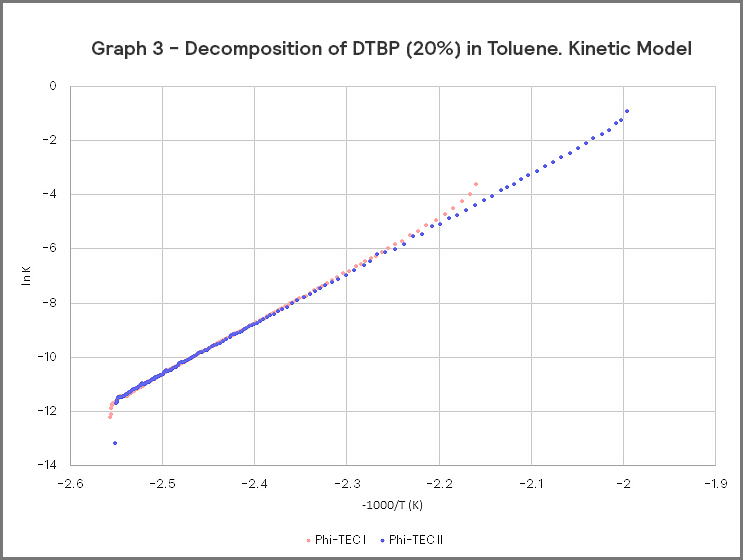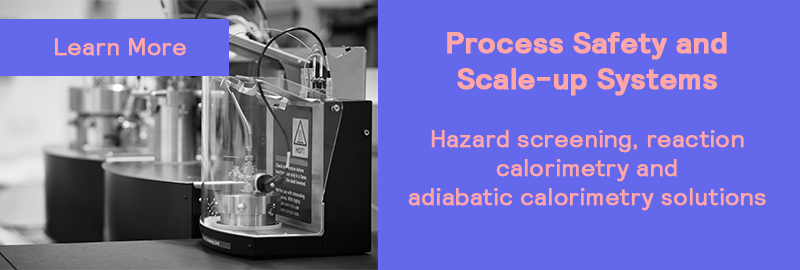A question that often crops up is what testing is best? Should we perform high Phi-factor or low Phi-factor tests (or in H.E.L terms, Phi-TEC I or Phi-TEC II)? Is one better than the other?

As is often the case, there is no straightforward answer and it will depend on the desired outcome of the test. Some testing procedures, such as vent sizing, need low Phi-factor tests. However, sometimes you will find that you will make similar conclusions of the test from high Phi-factor testing.

# High Phi-factor or low Phi-factor tests?Graph 1 shows a typical temperature-time plot for the decomposition of 20% DTBP (Di-tert-butyl peroxide) in toluene carried out in both a Phi-TEC I (Phi-factor = 2.04) and a Phi-TEC II (Phi-factor = 1.10).

The results show that the lower Phi-factor test proceeds at a faster rate, has a higher rate of temperature change, and a higher final temperature.
This data is more representative of the effects observed on a large scale, compared to a small sample, high Phi-factor factor test.

# The Phi-factor effectGraph 2 shows the respective self-heat rates of the two tests.
We often show this graph to demonstrate the effects of Phi-factor. The low Phi-factor test clearly has a higher peak, and the magnitude of the difference is clear. In this case, the low Phi-factor gives a rate of temperature change almost 16 times higher than the high Phi-factor test.

This graph is often an eye-opener for some. However, the data is typical and can be expected for many reactions.

There are two main reasons for the higher heating rate. The first is that due to the lower Phi-factor, less heat from the decomposition goes into heating the test cell.
This, in turn, means the temperature increases further (due to reaction kinetics, reactions progress faster at higher temperatures). The more dominant effect is simply that the temperature is much higher.If the kinetic plots of both tests are investigated, we get Graph 3.
What is apparent is that all the data points fall on a straight line. This is not surprising, as it is after all, the same reaction.
The main difference between the two sets of kinetic plots, is that the low Phi-factor test covers a larger temperature range.
This means the same kinetic model and parameters would be obtained from either test, and the data is comparable. If you are looking for kinetic data for TMR, SADT etc, calculations, then either high or low Phi-factor tests will suffice.

# .Conclusion – which is the best solution?

The question we initially asked is, which is best?

There is no straightforward answer, as both will provide significant information to allow hazard assessment and the preparation of a safety case.

A factor to consider, is that low Phi-factor tests could come at a premium. In terms of equipment cost, running costs, and indeed sample costs (due to a larger volume of sample being needed), low Phi-Factor tests generally have a higher operating cost. However, this is a trade-off with data that is very close to what would happen if a large scale reactor suffered a runaway reaction.

The nature of high Phi-factor testing dictates that lower temperatures, and therefore lower heating rates, and indeed the corresponding pressure and rate of pressure rise, are orders of magnitude lower than would be experienced on a larger scale.
As long these factors are taken into consideration, it is possible to obtain much of the data you need from a high Phi-factor test – onset data, kinetics, propensity for gas generation, and so on.

As previously mentioned, there are times when low Phi-factor tests are essential. This is mainly for relief line sizing and investigating worst-case scenarios.
These cannot be done from high Phi-factor tests. Of course, the other use is that the rate of change in both pressure and temperature can certainly be an eye-opener to non-specialists who would otherwise not appreciate the difference.